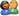登  录风  格绿草青青青青苹果蓝色经典佳人有约蓝色梦想蓝天白云蓝色狂想蓝色魅力魅力星空
 »  游客当前位置：  论坛首页 »  自由讨论区 »  Android, Python及开发编程讨论区 »总帖数 1 每页帖数 10 1/1页 1
0查看: 7091 | 回复: 0 主题： [转帖]JAVA经典算法几例（3）liulin_2 注册用户等级：少校 经验：1143 发帖：85 精华：5 注册：2012-12-10 状态：离线加好友发消息

 【程序 11】 题目：有1、2、3、4个数字，能组成多少个互不相同且无重复数字的三位数？都是多少？ 1. 程序分析：可填在百位、十位、个位的数字都是1、2、3、4。组成所有的排列后再去 掉不满足条件的排列。  public class Wanshu {      public static void main(String[] args)      {            int i=0;            int j=0;            int k=0;            int t=0;            for(i=1;i<=4;i++)            for(j=1;j<=4;j++)            for(k=1;k<=4;k++)            if(i!=j && j!=k && i!=k)            {t+=1;                  System.out.println(i*100+j*10+k);            }            System.out.println (t);      }}【程序 12】 题目：企业发放的奖金根据利润提成。利润(I)低于或等于10万元时，奖金可提10%；利润高于10万元，低于20万元时，低于10万元的部分按10%提成，高于10万元的部分，可可提成7.5%；20万到40万之间时，高于20万元的部分，可提成5%；40万到60万之间时高于40万元的部分，可提成3%；60万到100万之间时，高于60万元的部分，可提成1.5%，高于100万元时，超过100万元的部分按1%提成，从键盘输入当月利润I，求应发放奖金总数？ 1. 程序分析：请利用数轴来分界，定位。注意定义时需把奖金定义成长整型。  import java .util.*;public class test {      public static void main (String[]args){            double sum;// 声明要储存的变量应发的奖金             Scanner input =new Scanner (System.in);// 导入扫描器             System.out.print (" 输入当月利润");            double lirun=input .nextDouble();// 从控制台录入利润             if(lirun<=100000){                  sum=lirun*0.1;            }else if (lirun<=200000){                  sum=10000+lirun*0.075;            }else if (lirun<=400000){                  sum=17500+lirun*0.05;            }else if (lirun<=600000){                  sum=lirun*0.03;            }else if (lirun<=1000000){                  sum=lirun*0.015;            } else{            sum=lirun*0.01;      }      System.out.println(" 应发的奖金是"+sum);}}后面其他情况的代码可以由读者自行完善 .【程序 13】 题目：一个整数，它加上 100后是一个完全平方数，加上168又是一个完全平方数，请问该数是多少？ 1. 程序分析：在10万以内判断，先将该数加上100后再开方，再将该数加上268后再开方，如果开方后的结果满足如下条件，即是结果。请看具体分析：  public class test {      public static void main (String[]args){            long k=0;            for(k=1;k<=100000l;k++)            if(Math.floor(Math.sqrt(k+100))==Math.sqrt(k+100) && Math.floor(Math.sqrt(k+168))==Math.sqrt(k+168))            System.out.println(k);      }}【程序 14】 题目：输入某年某月某日，判断这一天是这一年的第几天？ 1. 程序分析：以3月5日为例，应该先把前两个月的加起来，然后再加上5天即本年的第几天，特殊情况，闰年且输入月份大于3时需考虑多加一天。  import java.util.*;public class test {      public static void main (String[]args){            int day=0;            int month=0;            int year=0;            int sum=0;            int leap;            System.out.print(" 请输入年,月,日\n");            Scanner input = new Scanner(System.in);            year=input.nextInt();            month=input.nextInt();            day=input.nextInt();            switch(month)            {                  case 1:                  sum=0;break;                  case 2:                  sum=31;break;                  case 3:                  sum=59;break;                  case 4:                  sum=90;break;                  case 5:                  sum=120;break;                  case 6:                  sum=151;break;                  case 7:                  sum=181;break;                  case 8:                  sum=212;break;                  case 9:                  sum=243;break;                  case 10:                  sum=273;break;                  case 11:                  sum=304;break;                  case 12:                  sum=334;break;                  default:                  System.out.println("data error");break;            }            sum=sum+day;            if(year%400==0||(year%4==0&&year%100!=0))            leap=1;            else            leap=0;            if(leap==1 && month>2)            sum++;            System.out.println("It is the the day:"+sum);      }}【程序 15】 题目：输入三个整数x,y,z，请把这三个数由小到大输出。 1. 程序分析：我们想办法把最小的数放到x上，先将x与y进行比较，如果x> y则将x与y的值进行交换，然后再用x与z进行比较，如果x> z则将x与z的值进行交换，这样能使x最小。  import java.util.*;public class test {      public static void main (String[]args){            int i=0;            int j=0;            int k=0;            int x=0;            System.out.print(" 请输入三个数\n");            Scanner input = new Scanner(System.in);            i=input.nextInt();            j=input.nextInt();            k=input.nextInt();            if(i>j)            {                  x=i;                  i=j;                  j=x;            }            if(i>k)            {                  x=i;                  i=k;                  k=x;            }            if(j>k)            {                  x=j;                  j=k;                  k=x;            }            System.out.println(i+", "+j+", "+k);      }}

 本版精华 热门帖子操作    引用/回复总帖数 1 每页帖数 10 1/1页 1请输入验证码：您需要登录后才可以回帖 登录 | 注册技术讨论